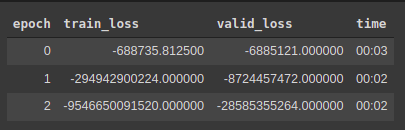# Insanely large train and validation loss

Problem: I can’t seem to get my network training properly. The losses are insanely high and the addition of an argument except ‘None’ to the ‘metric=’ keyword prevents training

I am trying to perform semantic segmentation on RGB images of cells. I am using a UNet structure and want to compare how different backbones/encoders (ResNetX, DenseNet etc…) perform.
Both the images (X, [3, 256, 256]) and the masks (y, [256, 256]) are uint8 in their raw form. The masks only contain 0 or 255 which corresponds to cell and not cell, respectively.

Dataset and loader:
dblock = DataBlock(blocks = (ImageBlock, MaskBlock),
get_items = get_image_files,
get_y= get_y_fn,
item_tfms = RandomCrop(INPUT_SHAPE),
batch_tfms = aug_transforms()
)
dset = dblock.datasets(rawPath)
dls = dblock.dataloaders(rawPath, bs=16)

Network:
encoder = nn.Sequential(*list(resnet18(pretrained=pretrained).children())[:-2])
resm = DynamicUnet(encoder, 1, (INPUT_SHAPE,INPUT_SHAPE))
resm.cuda()

Learner:
learn = Learner(dls, resm, loss_func=BCEWithLogitsLossFlat(), opt_func = Adam, metrics=accuracy)

When metrics = accuracy, I get this error message:
AssertionError: ==:
4096
1048576

I believe this is something to do with the flattening that the loss function does as 4096 = 16 * 256 = bs * image_side, and 1048576 = 256 * 256 * 16. However, I don’t understand why using the flat loss would mean I can’t use accuracy or other metrics.
I choseBCEWithLogitsLossFlat() as this is a binary task and the input is not one-hot encoded.

When metrics = None, I get this from the learn.fit_one_cycle() command.The more epochs I train for, the worse it gets.

Can anyone see where I am going wrong?